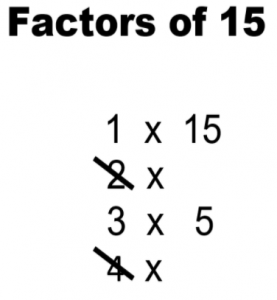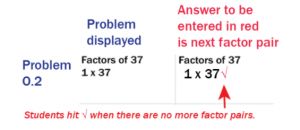## Rocket Math’s Foolproof Method to Finding Factors (A.K.A. Factor Pairs)

In order for a student to expand or reduce fractions or to add and subtract unlike fractions, they need to know how to find factors for each number in the fraction. Students may know multiplication facts, but still, find it difficult to come up with all the possible factor pairs for a given number. The key to helping students with this task is to teach students a systematic method of identifying all factor pairs and then committing both the process and some common numbers to memory.

Both the Rocket Math Online Game and Worksheet Program uses a foolproof, systematic method for teaching students how to find all the factors of a number. By reading this article, you’ll learn what factors are, how to help students find all the factors systematically, and how both the game and worksheets incorporate the method into each program.

## What are Factors of a Number

Factors of a number, such as 12, are pairs of whole numbers whose product is that number. For example, 2 x 6 = 12, 12 is the product, and the factor pair is 2 x 6. Other factor pairs for 12 include 1 x 12 and 3 x 4 because multiplied together, each of those factor pairs equals 12.

## Finding Factors: How do You Know When You Have Found all of them?

Identifying some of the factors of a target number isn’t hard, but knowing when you have accounted for ALL the factors can be hard for students. Rocket Math’s Worksheet Program helps students find and memorize all the factors for 22 common numbers. Watch this video to see how Dr. Don uses the worksheet program to teach students how to find all of a number’s factors in a systematic way.1. The best method for teaching students how to find factor pairs is to have them start at 1 and work their way up. Give your students a target number and ask them to put “1 x” below it. Let them fill in the right side with the number itself. We know that any number has one “factor pair” of 1 times itself.
2. Then ask them to move on to 2, and ask themselves, “What number times 2 equals the target number?” If the target number is even, there will be a number on the right-hand side of that pair. If the target number is odd (like 15), there won’t be, and the student should cross out the 2, as it is not a factor of the target number.
3. Next, the student moves on to 3. They ask themselves, “What number times 3 equals 15?” Because 3 x 5 equals 15, students write 5 down because that is the next factor pairing 15.
4. Next, students move on to 4. They ask themselves, “What number times 4 equals 15?” When students run across a number that is not a factor of the target number, they should cross it out. So they cross out the 4.
5. The next number is 5, but it is already showing on the right side of the factor pairs. When that happens, students can be sure they have found all the factor pairs of the target number.

In the end, students will be left with a list of factor pairs that multiply to create the target and a list of crossed-out numbers that are not factors.

## Rocket Math Online Game – Finding Factors & Primes Track

Building on what the Worksheet Program has to offer students, Learning Track #15 (Factors & Primes) of the Rocket Math Online Game helps students systematically identify, practice, and memorize factor pairs of every number from 2 through 40 and 42, 45, 48, 49, 50, 64, 72, 75, and 100.

### Students answer with the NEXT factor pair in order

Factors and Primes has a unique way of teaching students the factors. Students learn the factor pairs, in order, starting with 1 and itself. For example the factor pairs of 36, in order, are 1 x 36, 2 x 18, 3 x 12, 4 x 9 and 6 x 6.   Students are shown some factor pairs in the display (see problems displayed in N.1 through O.1). They must either enter the next factor pair in order or hit the checkmark if there are no more pairs. You can see the five problems that deal with the factors of 36 that students encounter in the Factors and Primes Learning Track.

When students see problem N.1 displaying “Factors of 36, 1 x 36” they learn that the next factor pair is 2 x 18, so they enter a 2, a 1, and an 8, and the game displays them as shown.  If the student does not enter the next correct factor pair, the game’s audio voice recording (called Mission Control) says, “The factors of 36, in order, are 1 times 36 then, 2 times 18. Go again.” The game then provides an opportunity to answer that problem again, twice, and then interspersed with other problems, twice more before the game considers that pair of factors learned.

When students see problem O.1 they see all the factor pairs of 36 displayed. They know that they have all the factor pairs because the same number is in the left-hand column and the right-hand column. Students are to hit the checkmark √, to indicate there are no more factor pairs. The game’s audio voice recording correction is, “There are no more factors of 36. Just hit the checkmark. Go again.”

### Prime numbersAny of the numbers that students learn are prime numbers will begin with the first factor pair of 1 times the number itself. For example, in problem, O.2 students see “Factors of 37. 1 x 37.”  Students are to hit the checkmark to indicate that a number is a prime number.  The game’s audio voice correction is, “37 is a prime number because its only factors are 1 times itself. Just hit the checkmark. Go again.”  In this manner, students learn all the prime numbers from 2 to 40 in this Learning Track.

Students should learn their multiplication and division facts first before working on Factors and Primes. Once those are mastered in the Online Game, students who are already trying to find the greatest common denominators in working with fractions the Factors & Primes Learning Track will help them greatly.  They will exit knowing the factor pairs of these most common numbers and they will find this work much easier.

## Make finding factors easier (and more fun!) for your students with Rocket Math

With Rocket Math’s Factor Worksheet and the Online Game, your students can say goodbye to slow progress and hit-and-miss strategies. The Worksheet and Online Game can be used separately or in tandem for extra practice.

Try Learning Track #15 (Factors & Primes) of the Rocket Math Online Game for Free!